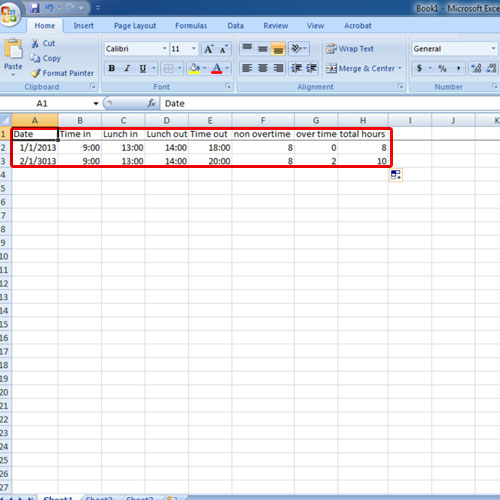## How to Make Hourly Work Time Sheet

In this tutorial, we will teach you how to make hourly work time sheets. People make hourly work time sheets to calculate the total hours worked. For the purpose of this tutorial, we will break the total number of hours into non-overtime hours and overtime hours.

Step 1 – Enter the headings

Follow this step by step guide to learn how to make a hourly work time sheet.
First of all, type in the fields that you want to include in your time sheet in an Excel workbook. For the purpose of this tutorial, we will be creating a basic hourly work time sheet which will calculate the total number of work hours. Simply enter the time in and time out for a person along with their lunch hours and the total number of hours will be calculated.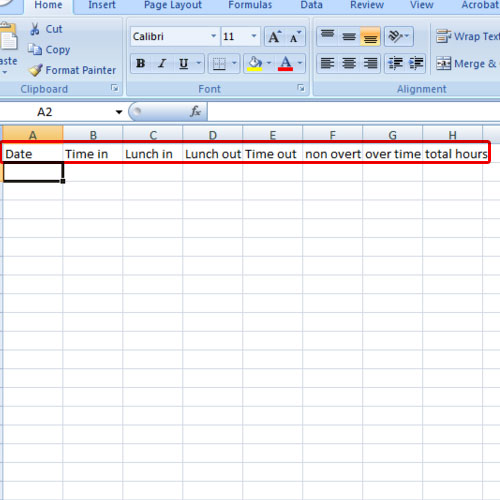Step 2 – Freeze top row

Once you have entered the headings for the work time sheet, go to view and freeze the top row where the headings are. Next, make sure the date field has the proper data type selected. For example in the time fields, make sure the time category is selected in the format cells window.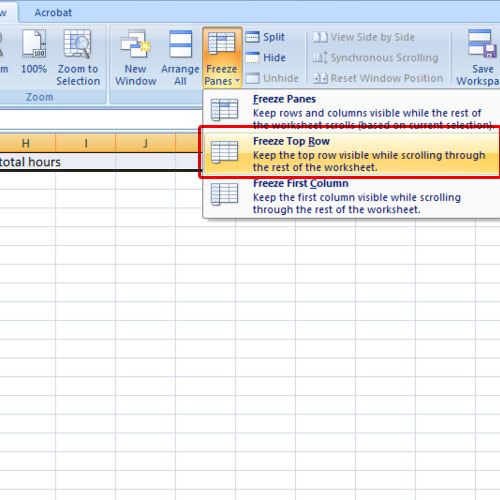Step 3 – Enter formulas

We will break the total hours into non overtime and overtime. We will consider 8 hours as the regular or non-overtime hours. We will place 8 under the non-overtime hour’s column. In the overtime column, we will enter the formula “=SUM(H2-8)”. H2 over here represents total hours.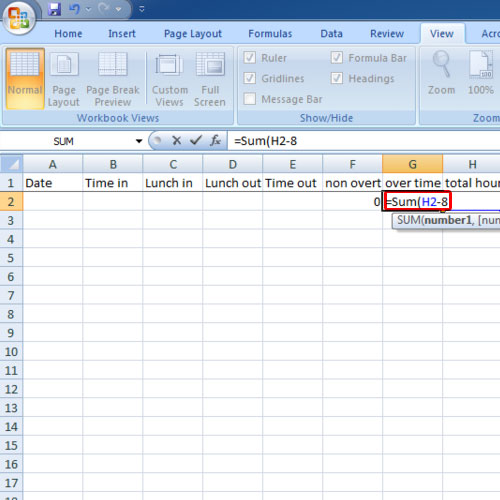Step 4 – Enter formula for total hours

Now enter the following formula under the total hour’s column:
=SUM((C2-B2)+(E2-D2))*24
You need to make sure the total hour’s format is number.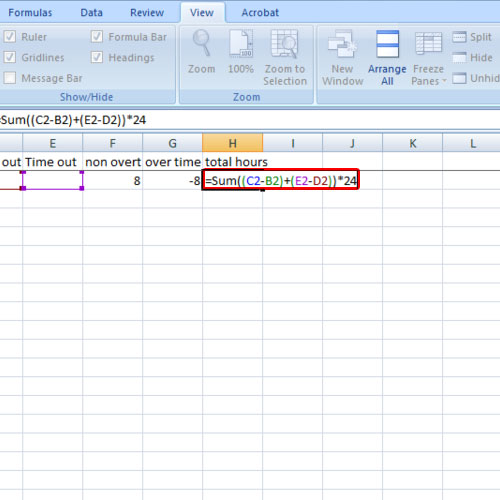Step 5 – Data Entry

Now fill in the data for a certain date. You will see that the total number of hours will be calculated automatically as per the formula. We will even make a case where there are overtime hours and you will see that they are even included in the total count.
In this manner, you can make hourly work time sheets.